# KSEEB SSLC Class 10 Maths Solutions Chapter 9 Polynomials Ex 9.1

In this chapter, we provide KSEEB SSLC Class 10 Maths Solutions Solutions Chapter 9 Polynomials Ex 9.1 for English medium students, Which will very helpful for every student in their exams. Students can download the latest KSEEB SSLC Class 10 Maths Solutions Solutions Chapter 9 Polynomials Ex 9.1 pdf, free KSEEB SSLC Class 10 Maths Solutions Solutions Chapter 9 Polynomials Ex 9.1 pdf download. Now you will get step by step solution to each question.

### Karnataka State Syllabus Class 10 Maths SolutionsChapter 9 Polynomials Ex 9.1

Question 1.
The graph of y = p(x) are given in the following figure, for some polynomials p(x). Find the number of zeros of p(x) in each case.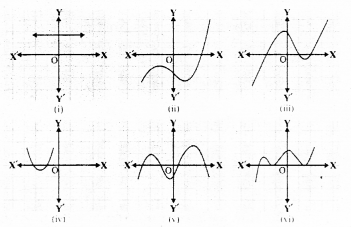Solution:
(i) Graph of y = p(x) will not intersects x-axis.
∴ No zero for this polynomial.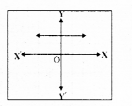(ii) Graph of y = p(x) intersects x-axis at only one point.
∴ Only one zero is there for this polynomial.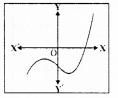(iii) y = p(x) intersects x-axis at 3 points.
∴ It has 3 zeroes.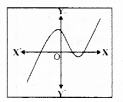(iv) y = p(x) intersects x-axis at 2 points.
∴ Two zeroes for this polynomial.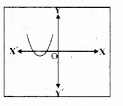(v) y = p(x) intersects x-axis at four (4) points.
∴ Four zeroes for this polynomial.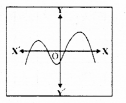(vi) y = p(x) intersects x-axis at three (3) points.
∴ This polynomial has 3 zeroes.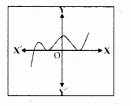All Chapter KSEEB Solutions For Class 10 Maths

—————————————————————————–

All Subject KSEEB Solutions For Class 10

*************************************************

I think you got complete solutions for this chapter. If You have any queries regarding this chapter, please comment on the below section our subject teacher will answer you. We tried our best to give complete solutions so you got good marks in your exam.

If these solutions have helped you, you can also share kseebsolutionsfor.com to your friends.

Best of Luck!!